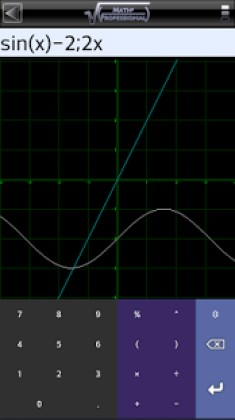# Math Professional 0.1.6 Apk patched latest

event_note09 Jan 2018

Math Professional 0.1.6 Apk patched latest is a Productivity Android app

Math Professional is a Productivity android app made by SMH17 that you can install on your android devices an enjoy !
Math Professional is the ultimate math tool for your mobile.

✓DOESN’T REQUIRE INTERNET CONNECTION

You can access almost all functions also without internet connection.

✓GRAPHIC CALCULATOR

Can perform all the functions of an advanced scientific calculator and is able to plot 2D and 3D functions, also provides numeric conversion octal, hexadecimal and binary forms, allows the derivative calculation, multi plotting and the direct plotting of the derivatives functions. Includes logarithmic functions, trigonometric and hyperbolic functions, allows for the calculation of the MCD, linear combinations and permutations, factorization and much more. See the help in the section for detailed information on how to invoke the functions and syntax notation to use.

✓EQUATION SOLVER

Allow to find the equation roots real and complex. Just set the degree of the equation and fill out the fields to get the result.

✓MATRIX SOLVER

This tool provide a powerful matrix solver. You can calculate inverse, transpose, the standard rank, exponentiation, break it down, finding the determinant, finding eigenvalues ​​and eigenvectors, calculate the product, addition, subtraction and multiplication of matrices and much more.

✓GEOMETRIC SOLVER

• Midpoint coordinates in 2D and 3D plane
• Distance between two points in 2D and 3D plane
• Linear interpolation
• Calculation of perimeter and area of plane figures
• Calculation of volume and surface of solids
• Perpendicular bisector
• Perpendicular distance
• Point slope straight line eq
• Segment coordinates of points
• Slope intercept straight line eq
• Vectors cartesian product
• Vectors scalar products

✓STATISTICAL TOOLS

• Autocorrelation
• CUSUM
• Cohen’s D
• Covariance
• Effect size
• Elliptic Gamma function
• Empiric rule
• F-Test
• Fibonacci
• Geometric mean
• Hankel function
• Inverse Gamma distribution
• Linear regression
• Logarithmic Gamma distribution
• Mean
• Median
• Mode
• Neumann function
• Percentile to Z-score
• Polygamma function
• Pooled variance
• Skewness
• Spherical Hankel function
• T-Test
• T-Test critical value
• Tukey post-hoc test
• Variance
• Z-score to percentile

✓PROBABILISTIC FUNCTIONS

• Bayesian inference
• Binomial distribution
• Cauchy-lorentzian distribution
• Expected value
• Exponential distribution
• Gumbel distribution
• Hypergeometric distribution
• Multinomial distribution
• Normal distribution
• Poisson distribution
• Probability density distribution
• Rayleigh distribution
• Venn conditional probability
• Weibull cumulative distribution

✓FINANCIAL TOOLS

Allows you to meet all major needs in financial calculation. The list of available features includes the calculation and or analysis of:
• Break even calculator
• Dividend payout ratio
• FCNR cash certificate issue price
• FCNR income deposit
• Individual retirement account (IRA)
• Interest rate calculator
• Inventory cost in both FIFO and LIFO mode
• Mortgage payment calculator
• Net Present Value calculator (NPV)
• Retirement calculator
• Return on investment (ROI)
• Total return
• Value Added Tax (VAT) calculator

✓EQUIVALENCE CALCULATOR

Allows you to convert and execute operations between different unit scales or monetary currencies. Thousands units are supported, just hold down the button unit to view the complete list sorted by category, or directly enter the name of the unit in the search box that will appear with a short click on the button unit. Scrolling the keyboard to the left, you can access additional commands. Are also permitted transactions between different units on condition that are compatible with each other.

…and much more is coming free for the users that purchase the app now.

The app is available in English and Italian, more translation and functions are coming. Stay tuned.

In case of problems or suggestion use the form on the site:Math Professional ApkMath Professional Apk

Whats New:
v0.1.6
-Various Code optimizations
-Bug fix
v0.1.4
-Improved matrix solver
v0.1.3
-Improvements and bug fix
v0.1.2
-Increased 3D rendering quality
-Improved GUI quality for various large screen devices
v0.1.0
-Equation Solver Improved
v0.0.9
-Added Net Present Value (NPV) calculation function
-Fixed various issues and bugs in financial, probabilistic and statistical tools
v0.0.8
-Fixed wrong input bug in various special calculation functions
-Improved VAT calc